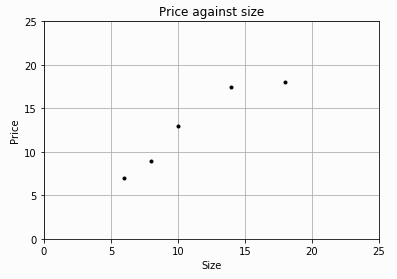## 2018/11/25

### Machine learning, scikit-learn 簡介

03 Machine learning, scikit-learn簡介

#### Machine learning （機器學習, ML）

• Supervised learning:利用已有的資料進行訓練並測試，完成的模型用以預測未見過的資料。若用以預測離散型資料則稱分類(Classification)， 若用以預測連續數值（如實數）則稱為回歸(Regression)。
• Unsupervised learning：將已有的資料進行叢集化(Clustering)，如何分成叢集和要分成幾個叢集是考量的重點。
• Reinforcement learning: 學習個體(Agent)不斷的自我進行學習，當成功達某項目標系統給予一定的獎賞(Reward),學習個體最終可完成使命。
Machine learning 重要觀念：
• 監督式學習將資料分成訓練集(Training set)和測試集(testing)，訓練集用以調整系統的參數而測試集用以測試系統的性能。 一般以可用資料的3/4量為訓練集而1/4為測試集。資料集的每一筆資料稱為實例 (Instance)，每一實例屬於某一標的 (target) 或類別(class)，資料裡有很多特徵(feature)， 此特徵就是資料的維度(Dimension)。
• Curse of dimensionality (維度的魔咒):當系統參數的數目增加，系統學習的複雜度呈指數成長。
• 當訓練的資料過多使得系統參數對訓練資料匹配非常良好，但當用測試資料測試時性能卻非常差，稱 Overfitting (過適配)。 當訓練資料不足或系統太簡單時，性能很差，稱Underfitting (欠適配)。
• Data normalization (資料正規化)：把資料的平均值調整為0而變異數調整為1。
• Feature engineeribg (特徵工程)：將資料進行必要的轉換，如把文字資料變成實數資料以利系統學習，為machine learning的一個次領域。

#### scikit-learn

• 提供supervised learning (監督式學習)、 unsupervised learning 所需各式演算法。
• Dimensionality Reduction, Feature extraction, Feature selection。
• Cross Validation, Parameter Tuning, Manifold Learning
• sklearn.datasets提供了乳腺癌、iris、加州房價等諸多開源的資料庫。
Simple linear regression 使用sklearn例子：

 實例 大小 價格 1 6 7 2 8 9 3 10 13 4 14 17.5 5 18 18

import numpy as np
import matplotlib.pyplot as plt
X = np.array([, , , , ])
y = [7, 9, 13, 17.5, 18]
plt.figure()
plt.title('Price against size')
plt.xlabel('Size')
plt.ylabel('Price')
plt.plot(X, y, 'k.')
plt.axis([0, 25, 0, 25])
plt.grid(True)
plt.show()簡單數據圖

from sklearn.linear_model import LinearRegression
model = LinearRegression()
model.fit(X, y)
test_obj = np.array([])
predicted_price = model.predict(test_obj)
print('A 12" 大小價格是: %.2f' % predicted_price)


$y=\alpha +\beta x$

print(model.coef_)
print(model.intercept_)


1.965517241379315

X = np.array([, , , , ])
y = [7, 9, 13, 17.5, 18]
x1 = np.arange(26)
y1 = 1.9655+ 0.976 *x1
plt.figure()
plt.title('Price against size')
plt.xlabel('Size')
plt.ylabel('Price')
plt.plot(X, y, 'k.', x1,y1, 'r')
plt.axis([0, 25, 0, 25])
plt.grid(True)
plt.show()列印直線

#### 進一步了解演算法

$\mbox{Var}(x)=\frac{\sum_{i=1}^{n}\ \ (x_i-\bar x)^2}{n-1}$

$\mbox{Cov}(x,y)=\frac{\sum_{i=1}^{n}\ (x_i-\bar x)(y_i-\bar y)}{n-1}$

$\beta=\frac{\mbox{Cov}(x,y)}{\mbox{Var}(x)}$

$\alpha =\bar y- \beta \bar x$

x_bar = X.mean()
print(x_bar)
variance = ((X - x_bar)**2).sum() / (X.shape - 1)
print(variance)


23.2

y = np.array([7, 9, 13, 17.5, 18])
y_bar = y.mean()
covariance = np.multiply((X - x_bar).transpose(), y - y_bar).sum() /(X.shape - 1)
print(covariance)
print(np.cov(X.transpose(), y))


22.650000000000002

beta = covariance / variance
alpha = y_bar - beta*x_bar
print(beta)
print(alpha)


#### 用Cost function 評估適度性

Cost function（成本函數）也稱用Lost function （損失函數）是用於定義量測的誤差，也就是量測所得的$\hat y$實資料裡的$y$值的差。此值可稱residuals（殘留）或 training error （訓練誤差）。模型建立好後以測試資料進行測試時所產生的誤差稱為prediction errors 或test errors。
RSS: residual sum of squares定義成$SS_{res}=\sum_{i=1}^{n}((y_i-f(x_i))^2)$，用於計算 R-squared。下列程式計算RSS,
print('Residual sum of squares: %.2f' % np.mean((model.predict(X - y) ** 2))


 實例 大小 價格 預測值 1 8 11 9.7759 2 9 8.5 10.7522 3 11 15 12.7048 4 16 18 17.5863 5 12 11 13.6811

$SS{tot}=\sum_{i=1}^{n} (y_i-\bar y)^2$

R-squared的定義是

$R^2=1-\frac{SS_{res}}{SS_{tot}}$

scikit-learn 裡的score method可用來計算R-squared。
import numpy as np
from sklearn.linear_model import LinearRegression
X_train = np.array([6, 8, 10, 14, 18]).reshape(-1, 1)
y_train = [7, 9, 13, 17.5, 18]
X_test = np.array([8, 9, 11, 16, 12]).reshape(-1, 1)
y_test = [11, 8.5, 15, 18, 11]
model = LinearRegression()
model.fit(X_train, y_train)
r_squared = model.score(X_test, y_test)
print(r_squared )